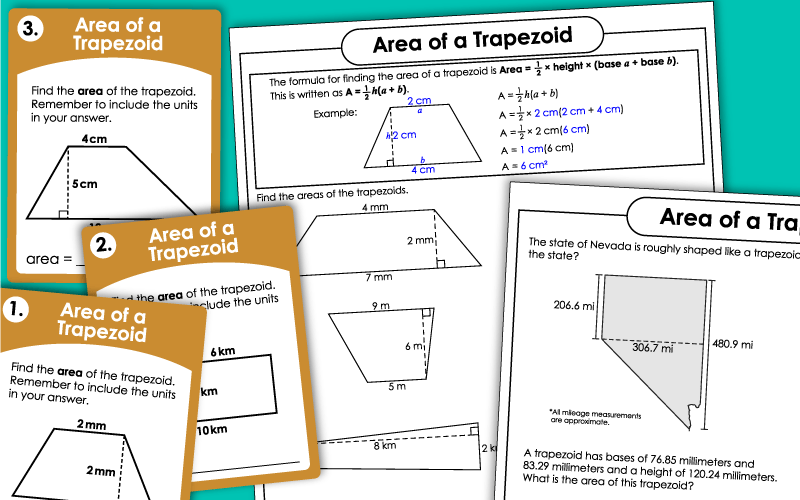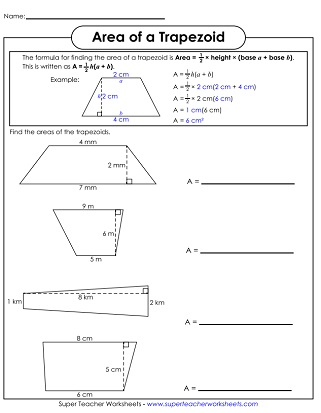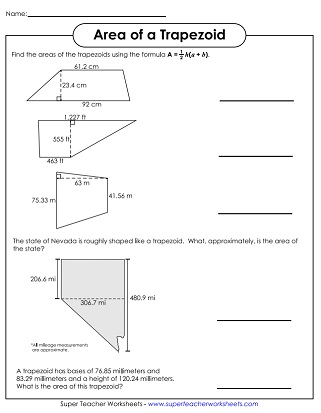# Area of Trapezoids

This page has a collection of printable worksheets for teaching students to calculate the areas of trapezoids.## Level: BasicSingle-Digit Measurements

Use the formula to calculate the areas of the trapezoids shown. This basic version contains small, whole number measurements.
Use the formula shown at the top to calculate the areas of the 9 trapezoids pictured.
Find both the perimeter and the area of each trapezoid shape shown.
Download a deck of 30 "area of a trapezoid" cards. Use them for peer study groups, classroom scavenger hunts, or small group instruction.

## Level: Intermediate2-Digit Measurements

Use the formula to calculate the area of the three trapezoids shown. In the intermediate-level worksheets, the measurements are all double-digit whole numbers.
This worksheets has 9 trapezoid models. Students can practice finding the area of each one.
These trapezoids have 2-digit measurements for each side. Find both the area and perimeter of each.

## Level: AdvancedIncludes Decimals & Fractions

Find the areas of five different trapezoids. This advanced-level worksheet includes 3-digit numbers and decimals.
First calculate the perimeter of each trapezoids by adding the lengths of the sides. Then find the area by multiplying the length of the base times the height.
More Area Worksheets

Find the areas of all types of shapes, including circles, rectangles, and triangles. There's a section for surface area worksheets too.

Geometry Worksheets

Link to printables on symmetry, tessellations, translation/rotation/reflection, polygons, plotting points, and more.

## Worksheet ImagesMy Account
Site Information